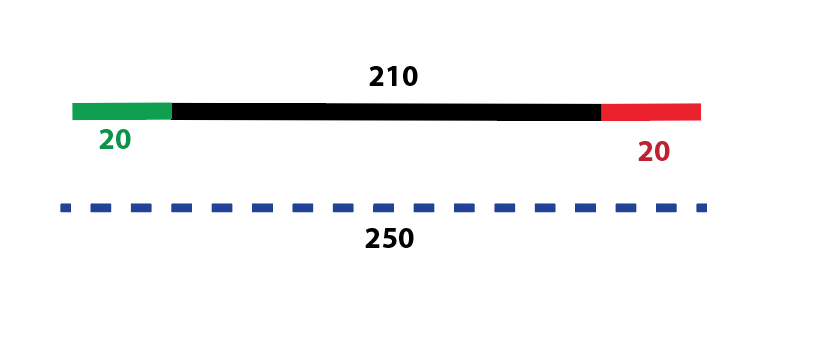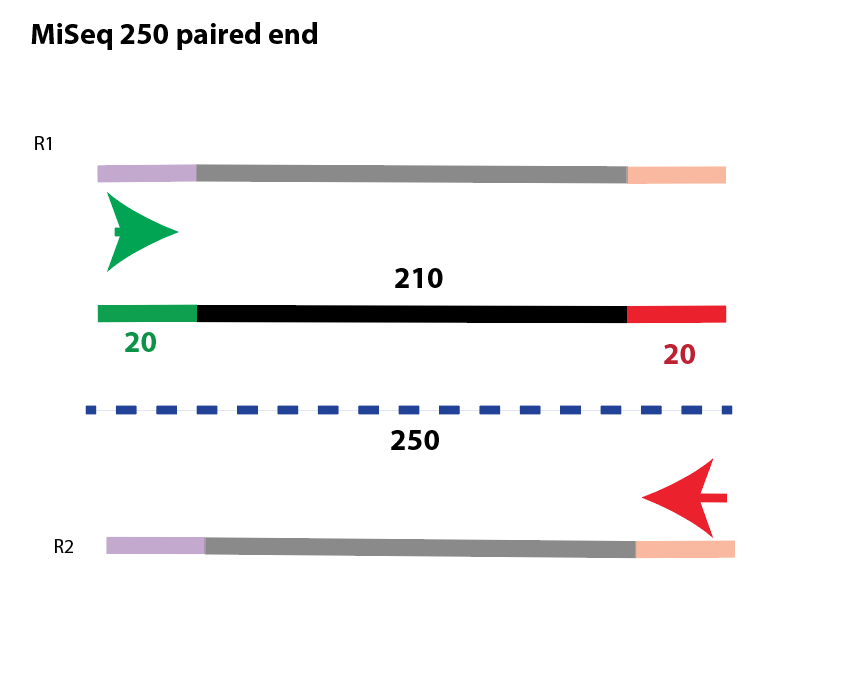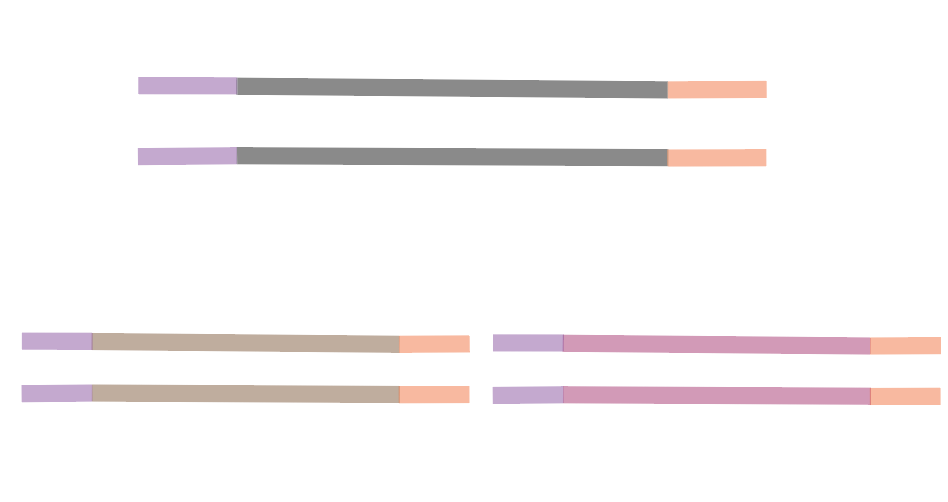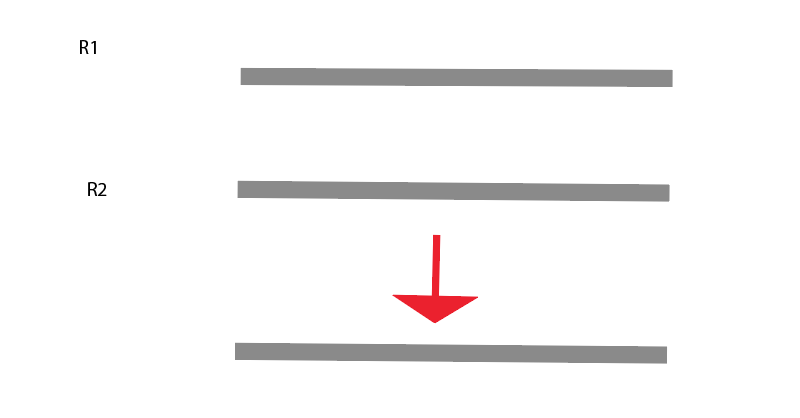{{ message }}

# Biancabrown / lab_presentation

Switch branches/tags
Nothing to show

Cannot retrieve contributors at this time
592 lines (354 sloc) 20.5 KB

## January 29, 2019

### This tutorial reviews the general workflow of a dada2 metabarcoding analysis pipeline, and several flavors of analysis that are commonly used in the Kartzinel lab at this time.

Traditionally, sequence reads are clustered into operational taxonomic units (OTUs) at a defined identity threshold to avoid sequencing errors generating spurious taxonomic units.

There have been numerous bioinformatic methods recently released that attempt to correct sequencing errors to determine real biological sequences at single nucleotide resolution by generating amplicon sequence variants (ASVs). A widely used pipeline for this type of analysis is called dada2.

Nearing et al.(2018) https://www.ncbi.nlm.nih.gov/pubmed/30123705

remember to cite

1. DADA2 is for modeling and correcting Illumina-sequenced amplicon errors
2. Infers sample sequences exactly and resolves differences of as little as 1 nucleotide.

Callahan at al. (2016) https://www.nature.com/articles/nmeth.3869

### This image represents a target amplicon locus with primers, which will be sequenced on an Illumina platform.primers are in green and red amplicon is in black Total length is 250 bp

### This image represents a demultiplexed sequence after Illumina sequencing.### This image represents multiple sequences within a single sample.When Illumina sequencing is complete, we recieve sample data in a "demultiplexed" state, which corresponds to one (in the case of single-end sequencing) or two (in the case of paired-end sequencing) fastq files. Below is an example of what a few lines of a fastq file look like.

``````Sample 1

@HWI-M02808:154:ANHE0:1:1101:17870:2090 1:N:0:AGGCATCTTACG
TACGGAGGATGCGAGCGTTATCCGGATTTATTGGGTTTAAAGGGTGCGTAGGCGGGTTGCTAAGTCAGTGGTAAAAGCGTGTGGCTCAACCATACCAAGCCATTGAAACTGGCGATCTTGAGTGTAAACGAGGTAGGCGGAATGTGACGTGTAGCGGTGAAATGCTTAGATATGTCACAGAACCCCGATTGCGAAGGCAGCTTACCAGCATACAACTGACGCTGAGGCACGAAAGCGTGGGTATCAAACA
+
@HWI-M02808:154:ANHE0:1:1101:17877:2107 1:N:0:AGGCATCTTACG
TACGGAGGATGCGAGCGTTATCCGGATTTATTGGGTTTAAAGGGTGCGTAGGCGGGTTGCTAAGTCAGTGGTAAAAGCGTGTGGCTCAACCATACCAAGCCATTGAAACTGGCGATCTTGAGTGTAAACGAGGTAGGCGGAATGTGACGTGTAGCGGTGAAATGCTTAGATATGTCACAGAACCCCGATTGCGAAGGCAGCTTACCAGCATACAACTGACGCTGAGGCACGAAAGCGTGGGGATCAAACA

``````
``````Sample 2

@HWI-M02808:154:ANHE0:1:1101:17870:2090 1:N:0:AGGCATCTTACG
TACGGAGGATGCGAGCGTTATCCGGATTTATTGGGTTTAAAGGGTGCGTAGGCGGGTTGCTAAGTCAGTGGTAAAAGCGTGTGGCTCAACCATACCAAGCCATTGAAACTGGCGATCTTGAGTGTAAACGAGGTAGGCGGAATGTGACGTGTAGCGGTGAAATGCTTAGATATGTCACAGAACCCCGATTGCGAAGGCAGCTTACCAGCATACAACTGACGCTGAGGCACGAAAGCGTGGGTATCAAACA
+
@HWI-M02808:154:ANHE0:1:1101:17877:2107 1:N:0:AGGCATCTTACG
TACGGAGGATGCGAGCGTTATCCGGATTTATTGGGTTTAAAGGGTGCGTAGGCGGGTTGCTAAGTCAGTGGTAAAAGCGTGTGGCTCAACCATACCAAGCCATTGAAACTGGCGATCTTGAGTGTAAACGAGGTAGGCGGAATGTGACGTGTAGCGGTGAAATGCTTAGATATGTCACAGAACCCCGATTGCGAAGGCAGCTTACCAGCATACAACTGACGCTGAGGCACGAAAGCGTGGGGATCAAACA

``````

Our general bioinformatic workflow uses dada2 to process the fastq files from each sequencing run. The pipeline needs to be applied to the set of samples from each sequencing library separately, and projects involving many sequencing libraries can be combined after this portion of the pipeline is complete. We begin by following this series of steps:

1. Summarize the sequence quality
2. Trim primers (there are multiple flavors for this step, depending on the length of the target sequences can also whether or not we used single- or paired-end sequencing to generate the data; more details below)
3. Remove sequences that are clearly errors (e.g., based on target length)
4. Check for errors using the dada2 function
5. Dereplicate the sequences
6. Remove chimeras
7. Assign taxonomy

A similar class of methods developed for 454-scale data was typically used to ‘denoise’ sequencing data prior to constructing OTUs (Quince et al., 2011), while new ASV methods are explicitly intended to replace OTUs as the atomic unit of analysis.

### R

``````library(dada2); packageVersion("dada2")
library(Rcpp); packageVersion("Rcpp")

path <- "fastq files"

# Forward and reverse fastq filenames have format: SAMPLENAME_R1_001.fastq and SAMPLENAME_R2_001.fastq
fnFs <- sort(list.files(path, pattern="R1_001.fastq", full.names = TRUE))
fnRs <- sort(list.files(path, pattern="R2_001.fastq", full.names = TRUE))
# Extract sample names, assuming filenames have format: SAMPLENAME_XXX.fastq
sample.names <- sapply(strsplit(basename(fnFs), "_"), `[`, 1)

#filt_path <- file.path(path, "filtered") # Place filtered files in filtered/ subdirectory
#filtFs <- file.path(filt_path, paste0(sample.names, "_F_filt.fastq.gz"))
#filtRs <- file.path(filt_path, paste0(sample.names, "_R_filt.fastq.gz"))

#out <- filterAndTrim(fnFs, filtFs, fnRs, filtRs,trimLeft = c(19, 20), truncLen=c(231,230),maxN=0, maxEE=c(2,2), truncQ=2, rm.phix=TRUE, compress=TRUE, multithread=TRUE)
#saveRDS(out, "out.rds")

#saveRDS(errF, "errF.rds")

#saveRDS(errR, "errR.rds")

#derepFs <- derepFastq(filtFs, verbose=TRUE)
#saveRDS(derepFs, "derepFs.rds")

#derepRs <- derepFastq(filtRs, verbose=TRUE)
#saveRDS(derepRs, "derepRs.rds")

#Name the derep-class objects by the sample names

#names(derepFs) <- sample.names

#names(derepRs) <- sample.names

#saveRDS(mergers, "mergers.rds")

#Inspect the merger data.frame from the first sample

#seqtab <- makeSequenceTable(mergers)
#saveRDS(seqtab, "seqtab.rds")

#saveRDS(seqtab.nochim, "seqtab.nochim.rds")

#saveRDS(taxa, "taxa.rds")

``````

Within the Kartzinel lab, we have many projects. Some projects are single-end reads, whereas others involve paired-end sequencing. Some projects have short and length-variable markers, whereas other projects use longer and single-length markers. The following lines show the key ways that we modify the dada2 pipeline to accomodate these different types of projects.

``````
We often use the 515/806 primers to amplify and sequence the 16S-V4 rRNA locus to analyze bacterial diversity and composition (i.e., microbiome). This marker is fixed-length and completely sequenced using paired-end sequencing with a XXX-cycle kit on the MiSeq. Therefore, we can truncate the sequence outputs at known positions by trimming the primers (trimLeft flag) and declaring the expected length of forward and reverse reads (truncLen flag).

out <- filterAndTrim(fnFs, filtFs, fnRs, filtRs,trimLeft = c(19, 20), truncLen=c(231,230),maxN=0, maxEE=c(2,2), truncQ=2, rm.phix=TRUE, compress=TRUE, multithread=TRUE)
saveRDS(out, "out.rds")

In many of our past and ongoing studies, we have generated data that require us to use a program like 'cutadapt' or 'NGSfilter' (in Obitools). Examples inlcude highly-plexed trnL-P6 experiments run on a HiSeq and demultiplexed using Obitools prior to quality filtering using dada2 and/or very long reads that are not completley sequenced using selected Illumina protocol. Here are examples of how we apply the filterAndTrim line in cases where primers have been removed previously, or cannot be removed using the trimLeft flag as described above.

This is an example used for trnL-P6, single-end sequencing data, with primers removed previously using the Obitools function 'NGSfilter'

out<-filterAndTrim(file.path(path,fns), file.path(filtpath,fns),maxN=0, maxEE=1, truncQ=2, rm.phix=TRUE,compress=TRUE, verbose=TRUE, multithread=TRUE)

This is an example used for trnL-P6, paired-end sequencing data, with primers removed previously using the 'cutadapt' function.

out <- filterAndTrim(fnFs, filtFs, fnRs, filtRs, maxN=0, maxEE=c(2,2), truncQ=2, minLen=8, rm.phix=TRUE, compress=TRUE, multithread=TRUE)

This is an example used for COI, paired-end sequencing data, with primers removed previously using the 'cutadapt' function, because the paired-end sequences are shorter than required to sequence both the forward and reverse reads in their entirety (i.e., spanning both forward and reverse primers on both strands, as detailed in the figures below).

out <- filterAndTrim(cutFs, filtFs, cutRs, filtRs, maxN = 0, maxEE = c(2, 2),
truncQ = 2, minLen = 50, rm.phix = TRUE, compress = TRUE, multithread = TRUE)

``````

Recapping the main reasons that we modify the filterAndTrim step to match common protocols used in the lab:

1. There is variation in the size of our amplicons
2. We have used multiple sequencing library strategies, which place different technical requirements on when and how we choose remove our primers from the sequence data. NB: dada2 REQUIRES that primers be removed prior to running the denoising scripts.

###Above example``````trim left =c(20, 20)

Remove primers from the beginning of the left forward (20) and reverse (20) sequences.

TruncLen=c(210,210)
remove sequences that are shorter than those length

``````###Example Number 2

Image if we had a 300 bp sequences

We are still doing Miseq 250 paired end

``````trim left =c(25,25)

#Trim forward primers and reverse primer. However, it will assume that each sequence has a forward and reverse primer

``````So we will get odd overlaps etc.

``````
path <- "files"
list.files(path)

fnFs <- sort(list.files(path, pattern="R1_001.fastq", full.names = TRUE))
fnRs <- sort(list.files(path, pattern="R2_001.fastq", full.names = TRUE))

FWD <- "GGWACWGGWTGAACWGTWTAYCCYCC"
REV <- "TANACYTCNGGRTGNCCRAARAAYCA"

allOrients <- function(primer) {
# Create all orientations of the input sequence
require(Biostrings)
dna <- DNAString(primer)  # The Biostrings works w/ DNAString objects rather than character vectors
orients <- c(Forward = dna, Complement = complement(dna), Reverse = reverse(dna),
RevComp = reverseComplement(dna))
return(sapply(orients, toString))  # Convert back to character vector
}

FWD.orients <- allOrients(FWD)
REV.orients <- allOrients(REV)

primerHits <- function(primer, fn) {
# Counts number of reads in which the primer is found
return(sum(nhits > 0))
}
rbind(FWD.ForwardReads = sapply(FWD.orients, primerHits, fn = fnFs[]),
FWD.ReverseReads = sapply(FWD.orients, primerHits, fn = fnRs[]),
REV.ForwardReads = sapply(REV.orients, primerHits, fn = fnFs[]),
REV.ReverseReads = sapply(REV.orients, primerHits, fn = fnRs[]))
``````

``````
#!/bin/bash

#SBATCH -n 8
#SBATCH --mem=100G
#SBATCH -t 2-00:00:00
#SBATCH --mail-type=ALL

#unzip

gunzip *.fastq.gz

#cut adapt to remove forward, reverse and their reverse complements.

for i in *_R1_001.fastq;

do
SAMPLE=\$(echo \${i} | sed "s/_R1_\001\.fastq//")
echo \${SAMPLE}_R1_001.fastq \${SAMPLE}_R2_001.fastq
cutadapt -a GGGCAATCCTGAGCCAA -g TTGGCTCAGGATTGCCC -A CCATTGAGTCTCTGCACCTATC -G GATAGGTGCAGAGACTCAATGG -o  \${SAMPLE}_trimmed_R1_001.fastq -p \${SAMPLE}_trimmed_R2_001.fastq  \${SAMPLE}_R1_001.fastq  \${SAMPLE}_R2_001.fastq

done

``````

If running it on your computer, you can use Brian's script with some modification:

``````
path <- "file"
list.files(path)

fnFs <- sort(list.files(path, pattern="R1_001.fastq", full.names = TRUE))
fnRs <- sort(list.files(path, pattern="R2_001.fastq", full.names = TRUE))

FWD <- "GGWACWGGWTGAACWGTWTAYCCYCC"
REV <- "TANACYTCNGGRTGNCCRAARAAYCA"

allOrients <- function(primer) {
# Create all orientations of the input sequence
require(Biostrings)
dna <- DNAString(primer)  # The Biostrings works w/ DNAString objects rather than character vectors
orients <- c(Forward = dna, Complement = complement(dna), Reverse = reverse(dna),
RevComp = reverseComplement(dna))
return(sapply(orients, toString))  # Convert back to character vector
}

FWD.orients <- allOrients(FWD)
REV.orients <- allOrients(REV)

primerHits <- function(primer, fn) {
# Counts number of reads in which the primer is found
return(sum(nhits > 0))
}
rbind(FWD.ForwardReads = sapply(FWD.orients, primerHits, fn = fnFs[]),
FWD.ReverseReads = sapply(FWD.orients, primerHits, fn = fnRs[]),
REV.ForwardReads = sapply(REV.orients, primerHits, fn = fnFs[]),
REV.ReverseReads = sapply(REV.orients, primerHits, fn = fnRs[]))
``````
``````
rm(list = ls())

library("plyr")
library("ggplot2")
library("phyloseq")
devtools::install_github("GuillemSalazar/FastaUtils")
require(FastaUtils)
library(Biostrings)
``````

``````path.cut <- "cutadapt"
list.files(path.cut)
cutFs <- sort(list.files(path.cut, pattern="R1_001.fastq", full.names = TRUE))
cutRs <- sort(list.files(path.cut, pattern="R2_001.fastq", full.names = TRUE))

sample.names <- sapply(strsplit(basename(cutFs), "_"), `[`, 1)

filtFs <- file.path(path.cut, "filtered", basename(cutFs))
filtRs <- file.path(path.cut, "filtered", basename(cutRs))

out <- filterAndTrim(cutFs, filtFs, cutRs, filtRs, maxN = 0, maxEE = c(2, 2),
truncQ = 2, minLen = 50, rm.phix = TRUE, compress = TRUE, multithread = TRUE)

derepFs <- derepFastq(filtFs, verbose=TRUE)
derepRs <- derepFastq(filtRs, verbose=TRUE)

names(derepFs) <- sample.names
names(derepRs) <- sample.names

seqtab <- makeSequenceTable(mergers)

getN <- function(x) sum(getUniques(x))
colnames(track) <- c("input", "filtered", "denoisedF", "denoisedR", "merged", "nonchim")
rownames(track) <- sample.names

barplot(track[,6], cex.names=0.5, las=2)

setwd("~/Desktop")
``````

## If running on oscar then we you need to do the following steps:

1. Write a R script dada2
2. Write a bash script and include the cutadapt code and dada2 code within that R script.
3. Call call bash script

###Structure of R script

R script are written in R

``````#!/usr/bin/env Rscript

``````

### Other things I will like to point out using our dada2 rscript

``````#!/usr/bin/env Rscript

library(Rcpp); packageVersion("Rcpp")

path <- "fast q"

# Forward and reverse fastq filenames have format: SAMPLENAME_R1_001.fastq and SAMPLENAME_R2_001.fastq
fnFs <- sort(list.files(path, pattern="R1_001.fastq", full.names = TRUE))
fnRs <- sort(list.files(path, pattern="R2_001.fastq", full.names = TRUE))
# Extract sample names, assuming filenames have format: SAMPLENAME_XXX.fastq
sample.names <- sapply(strsplit(basename(fnFs), "_"), `[`, 1)

filt_path <- file.path(path, "filtered") # Place filtered files in filtered/ subdirectory
filtFs <- file.path(filt_path, paste0(sample.names, "_F_filt.fastq.gz"))
filtRs <- file.path(filt_path, paste0(sample.names, "_R_filt.fastq.gz"))

out <- filterAndTrim(fnFs, filtFs, fnRs, filtRs,trimLeft = c(19, 20), truncLen=c(231,230),maxN=0, maxEE=c(2,2), truncQ=2, rm.phix=TRUE, compress=TRUE, multithread=TRUE)
saveRDS(out, "out.rds")

saveRDS(errF, "errF.rds")

saveRDS(errR, "errR.rds")

derepFs <- derepFastq(filtFs, verbose=TRUE)
saveRDS(derepFs, "derepFs.rds")

derepRs <- derepFastq(filtRs, verbose=TRUE)
saveRDS(derepRs, "derepRs.rds")

#Name the derep-class objects by the sample names

names(derepFs) <- sample.names

names(derepRs) <- sample.names

saveRDS(mergers, "mergers.rds")

#Inspect the merger data.frame from the first sample

seqtab <- makeSequenceTable(mergers)
saveRDS(seqtab, "seqtab.rds")

saveRDS(seqtab.nochim, "seqtab.nochim.rds")

saveRDS(taxa, "taxa.rds")

``````

saveRDS(errF, "errF.rds")

In case your program crash you can start again from that point.

1. Parts of the script that can be run in parallel:

## After writing R script, you write a bash script. If we were only going to call the bash script

General Structure of a bash script

``````#!/bin/bash

#SBATCH -J job_name
#SBATCH -o out
#SBATCH -e error
#SBATCH -n 8
#SBATCH --mem=100G
#SBATCH -t 2-00:00:00
#SBATCH --mail-type=ALL

blah blah code

``````

### Patrick's Example

``````
#!/bin/bash

#SBATCH -n 8
#SBATCH --mem=100G
#SBATCH -t 2-00:00:00
#SBATCH --mail-type=ALL

#unzip

gunzip *.fastq.gz

#cut adapt to remove forward, reverse and their reverse complements.

for i in *_R1_001.fastq;

do
SAMPLE=\$(echo \${i} | sed "s/_R1_\001\.fastq//")
echo \${SAMPLE}_R1_001.fastq \${SAMPLE}_R2_001.fastq
cutadapt -a GGGCAATCCTGAGCCAA -g TTGGCTCAGGATTGCCC -A CCATTGAGTCTCTGCACCTATC -G GATAGGTGCAGAGACTCAATGG -o  \${SAMPLE}_trimmed_R1_001.fastq -p \${SAMPLE}_trimmed_R2_001.fastq  \${SAMPLE}_R1_001.fastq  \${SAMPLE}_R2_001.fastq

done

#run dada2 script on trimmed sequences